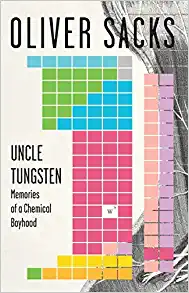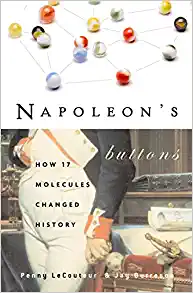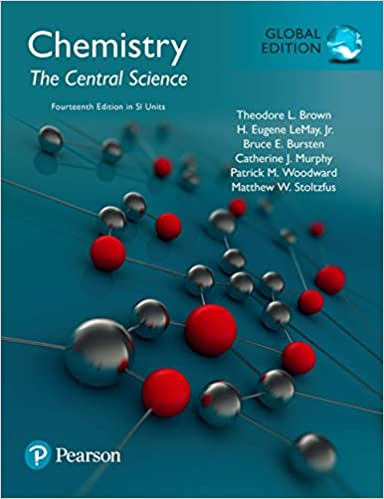# Percent Composition

The Percent Composition of a compound isn't something you can just figure out in your head. For instance, just because water is made up of one oxygen atom and two hydrogen atoms, that doesn't mean you can say water is 33% oxygen and 67% hydrogen. To determine percent composition by mass, you need to refer to a Periodic Table so you can see what each kind of atom weighs. With a little math, you'll be able to see that water is 88.81% oxygen by mass, and 11.19% hydrogen by mass. In this video, we work through two examples in full. We'll be your very own homework tutor for chemistry!

The Percent Composition of a compound isn't something you can just figure out in your head. For instance, just because water is made up of one oxygen atom and two hydrogen atoms, that doesn't mean you can say water is 33% oxygen and 67% hydrogen.

The percent composition is the percentage by mass of each element in a compound. It is calculated by dividing the mass of each element in the compound by the total mass of the compound, and expressing the result as a percentage.

To determine percent composition by mass, you need to refer to a Periodic Table so you can see what each kind of atom weighs. With a little math, you'll be able to see that water is 88.81% oxygen by mass, and 11.19% hydrogen by mass.

This video has 2 examples worked in full.

A practice test about Limiting Reactants, Percent Composition, and Stoichiometry with a full answer key is available for purchase in our digital store.

Video: Percent Composition